### Polynomial approximation in the $L^\infty$ norm

Given a continuous real-valued function $f$ on $[a,b]$, finding the best polynomial approximant $p_\infty$ to $f$ in the $L^\infty$-norm $\mbox{min}_{p\in\mathcal{P}_n}|f-p|_{L^{\infty}}$ is known as the minimax (or sometimes Chebyshev) approximation problem. These approximations can be computed by the Chebfun minimax command. Let's revisit the Chebfun example https://www.chebfun.org/examples/approx/ResolutionWiggly.html and compute a best polynomial approximant of degree 100:

dom = [0 14]; deg = 100;
f = chebfun(@(x) sin(x)^2 + sin(x^2), dom);
pinf = minimax(f, deg, 'tol', 1e-8);
plot([f pinf]), ylim([-3 3]), grid on
title('f and Linfty approximant')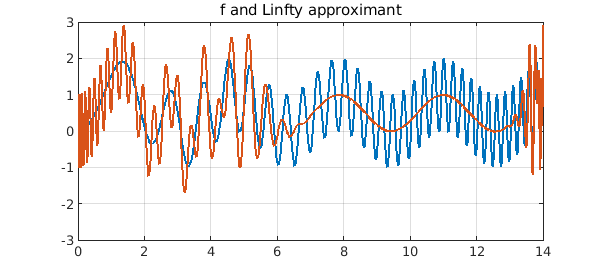The error $f-p_\infty$ exhibits the beautiful equioscillation phenomenon:

plot(f-pinf,'k'), ylim([-3 3]), grid on
title('error of Linfty approximant')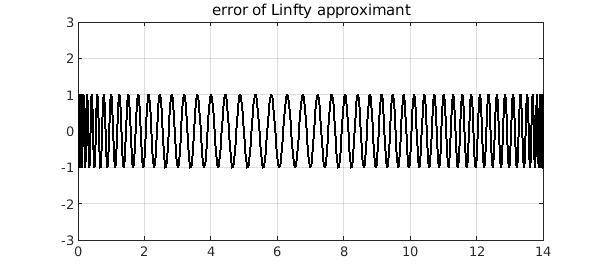### Polynomial approximation in the $L^2$ norm

The best polynomial approximant to $f$ in the $L^2$-norm is easier to compute as it is the orthogonal projection of $f$ onto the space of polynomials of degree $n$. In Chebfun, one can use the polyfit command:

p2 = polyfit(f, deg);
plot([f p2]), ylim([-3 3]), grid on
title('f and L2 approximant')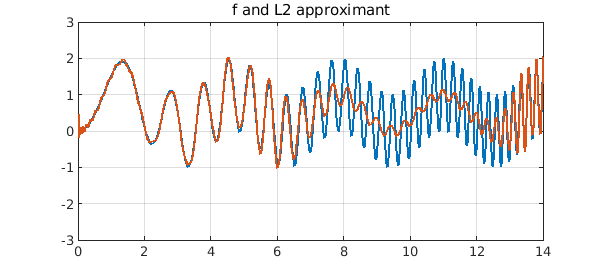The error curve is strikingly different. Of course it is slightly larger at its largest, but not by much.

plot(f-p2,'k'), ylim([-3 3]), grid on
title('error of L2 approximant')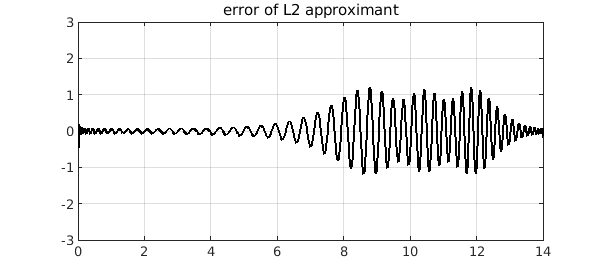### Polynomial approximation in the $L^1$ norm

Recently, we added a Chebfun polyfitL1 command to compute best polynomial approximants in the $L^1$-norm. (See the book by Pinkus for a survey of this subject .) Compressed sensing has made the $L^1$ norm an important tool in signal processing as it can promote sparsity in the solution or residual. A Newton-based algorithm proposed by Watson  is known to converge, under some assumptions, and this is the basis of polyfitL1.

p1 = polyfitL1(f, deg);
plot([f p1]), ylim([-3 3]), grid on
title('f and L1 approximant')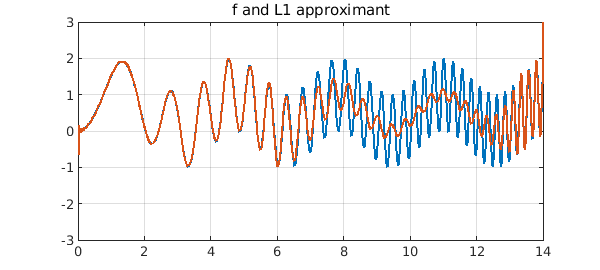Here is the error curve for our example. At first glance it looks like the $L^2$ case, but it is more strongly localized.

plot(f-p1,'k'), ylim([-3 3]), grid on
title('error of L1 approximant')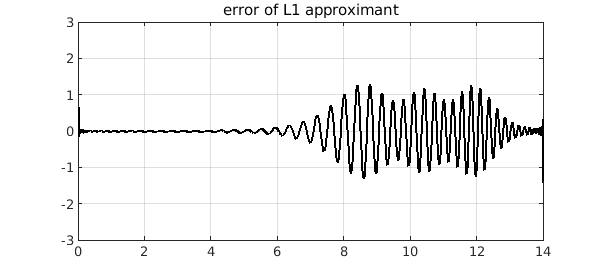### Another example

Let's do another example, following the example of Myth 3 of , the approximation of $|x-1/4|$ on $[-1,1]$ by a polynomial of degree $80$. This time we just plot the errors:

x = chebfun('x'); f = abs(x-1/4);
deg = 80;
pinf = minimax(f, deg);
plot(f-pinf,'k'), ylim(1e-2*[-1 1]), grid on
title('Linf error'), snapnow
p2 = polyfit(f, deg);
plot(f-p2,'k'), ylim(1e-2*[-1 1]), grid on
title('L2 error'), snapnow
p1 = polyfitL1(f, deg);
plot(f-p1,'k'), ylim(1e-2*[-1 1]), grid on
title('L1 error')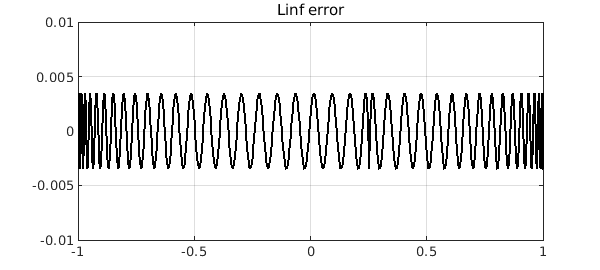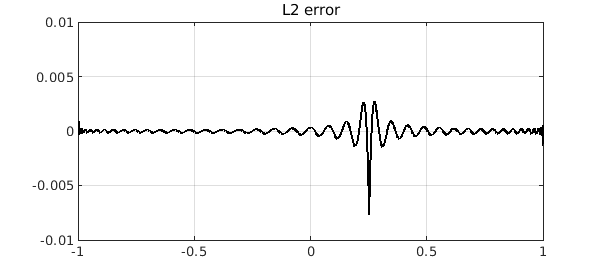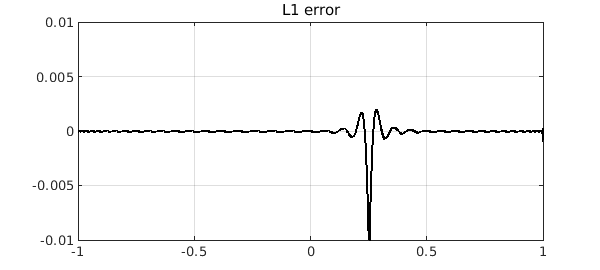Again, we see that the best $L^1$ polynomial approximant has a far more localized error. This is a typical phenomenon that is explained in~. To see more, we zoom the y axis by a factor of 100. There is much to be learned here!

ylim(1e-4*[-1 1])
title('closeup')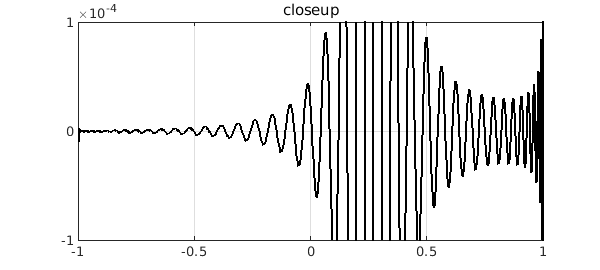### A word on the algorithm for polyfitL1

In , it is recommended that Watson's algorithm should be used in conjunction with linear programming problems and a refinement step. These additional algorithmic details can significantly speed up the computation. However, MATLAB's linear programming commands are in a toolbox, so we have avoided these steps in keeping with the Chebfun policy of just relying on core MATLAB.

 Y. Nakatsukasa and A. Townsend, Error localization of best L1 polynomial approximants, arXiv:1902.02664, 2019.

 A. M. Pinkus, On L1-approximation, Cambridge University Press, 1989.

 L. N. Trefethen, Six myths of polynomial interpolation and quadrature, appendix of Approximation Theory and Approximation Practice, SIAM, 2013.

 G. A. Watson. An algorithm for linear L1 approximation of continuous functions, IMA J. Numer. Anal., 1 (1981), 157--167.